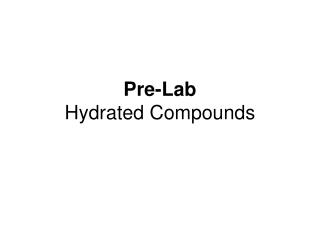DownloadDownload PresentationPre-Lab Hydrated Compounds

# Pre-Lab Hydrated Compounds

Télécharger la présentation## Pre-Lab Hydrated Compounds

- - - - - - - - - - - - - - - - - - - - - - - - - - - E N D - - - - - - - - - - - - - - - - - - - - - - - - - - -
##### Presentation Transcript

1. Pre-LabHydrated Compounds

2. Answer the following. Where appropriate answer in complete sentences. If a calculation is required show all your work. 1. Define: • hydrated compounds b) anhydrous compounds 2. What is the purpose of the desiccator? The purpose of the desiccator is to remove water from the hydrated compound after heating, leaving behind the anhydrous compound.

3. 3. Answer the following questions about the compound CoCl2 6 H2O • What does the dot mean? The dot gives the mole ratio of the anhydrous compound to water. b) What is the mole ratio of cobalt (II) chloride to water? c) What is the molar mass of CoCl2? d) What is the molar mass of 6 H2O? e) What is the molar mass of CoCl2 6 H2O? • What is the theoretical percentage of water in CoCl2 6 H2O? water mass x 100 hydrate mass

4. 4. Sample data table. Complete this table. Show calculations under the table. = #2 - #1 = #3 - #1 = #2a - #3b = #3c / #2a * 100 = #3b / molar mass = #3c / molar mass = #3e/smallest mol : #3f/smallest mol = write formula with mole ratio #s as coefficients

5. 5. A 140.5-g sample of NiSO4•XH2O is heated until no further decrease in mass. The mass of the anhydrous salt is 77.5 g. Find the coefficient for water in the formula of this hydrate of nickel (II) sulfate. • Steps • Find g H2O • Convert anhydrous g  mol • and H2O g  mol • Divide both by smallest • = whole # coeff.